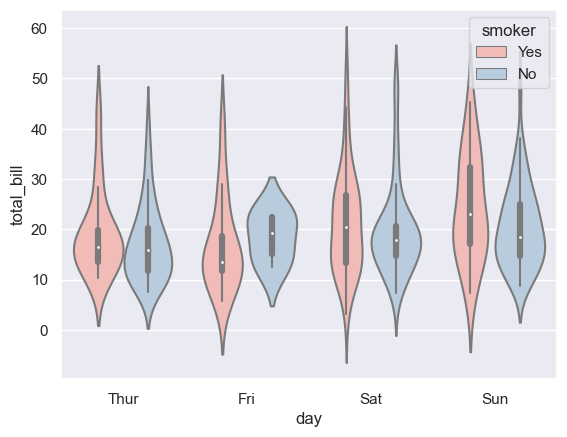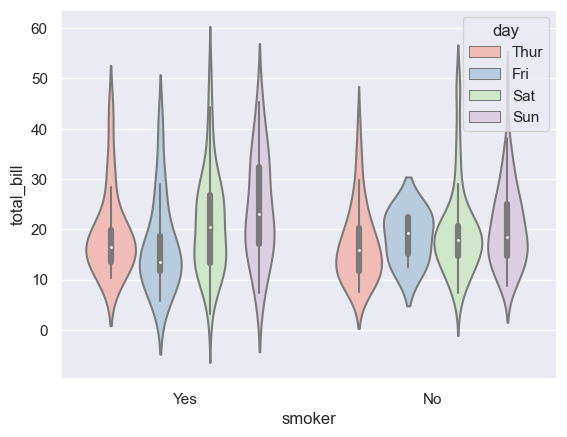## Libraries

First, you need to install the following librairies:

``````# libraries
import seaborn as sns
import matplotlib.pyplot as plt``````

## Dataset

The dataset we will use is the tips dataset, which we can easily download with seaborn:

In this dataset each row is a tip. For each tip we know the amount of the bill (`total_bill`) and several information like the day of the week (`day`) and if the customer was a smoker or not (`smoker`)

``df = sns.load_dataset('tips')``

## Grouped violin plot

In order to create a violin plot with seaborn, we need to add to the `hue` argument the name of the categorical variable that will separate our subgroups.

``````# set a grey background (use sns.set_theme() if seaborn version 0.11.0 or above)
sns.set(style="darkgrid")

# Grouped violinplot
sns.violinplot(x="day", y="total_bill", hue="smoker", data=df, palette="Pastel1")
plt.show()``````## Inverting categorical variables

A potentially relevant thing to do is to invert the categorical variable on the x-axis and the one in the `hue` argument. The output graph will be quite different, and may make it easier to understand the distribution of the underlying variables.

``````# set a grey background (use sns.set_theme() if seaborn version 0.11.0 or above)
sns.set(style="darkgrid")

# Grouped violinplot
sns.violinplot(x="smoker", y="total_bill", hue="day", data=df, palette="Pastel1")
plt.show()``````## Going further

This post explains how to create a grouped violin plot with seaborn.

For more examples of how to create or customize your violin plots, check the violin plot section. You may also be interested in how to customize a violin plot.

## Contact & Edit

👋 This document is a work by Yan Holtz. You can contribute on github, send me a feedback on twitter or subscribe to the newsletter to know when new examples are published! 🔥